• 问答
• 技术
• 实践
• 资源

## 目录

• 图像卷积
• 图像梯度
• 边缘提取

• 1. Prewitt算子
• 2. Sobel算子
• 3. Laplacian算子
• 平滑去噪

• 1. 高斯滤波
• 2. 均值滤波
• 3. 中值滤波

## 一、图像卷积

卷积(Convolution)：卷积运算就是对于图像 f(x,y) 中的每个像素，将其作为中心像素，计算它及其邻域像素和kernel核 w(a,b)对应位置元素的乘积，然后把结果相加到一起，得到的值就作为该中心像素的新值，这样就完成了一次卷积运算。然后将kernel向下或向左平移一位继续计算每个像素的新值，直到遍历完整个图像。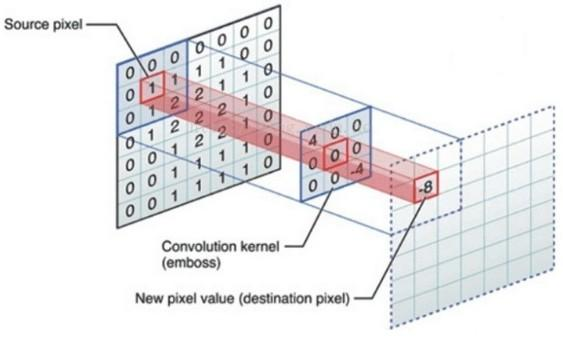$$f * w = \sum\limits_{(a,\ b) \in w \ (x-a,\ y-b) \in f} f(x-a,y-b)\ w(a,b)$$

$$f \otimes w = \sum\limits_{(a,\ b) \in w \ (x+a,\ y+b) \in f} f(x+a,y+b)\ w(a,b)$$

1. 最外面的一圈像素不进行计算，如果图像非常大，丢掉图像边缘的一圈对结果影响不大。
2. 更好的办法是人为地在图像四周插入一圈像素（比如最近邻插值）。

## 二、图像梯度

$$\nabla f=\begin{bmatrix} G{x} \ G{y} \end{bmatrix} = \begin{bmatrix} \dfrac{\partial f(x,y)}{\partial x} \ \dfrac{\partial f(x,y)}{\partial y} \end{bmatrix}$$

$$mag(\nabla f) = \sqrt{G{y}^{2} + G{x}^{2}}$$

$$\theta = \operatorname{arctan} \left (\dfrac{G{y}}{G{x}} \right)$$

$$\dfrac {\partial f}{\partial x} = f(x+1,\ y) - f(x,\ y) \ \dfrac {\partial f}{\partial y} = f(x,\ y+1) - f(x,\ y)$$

$$\dfrac {\partial f^2}{\partial x^2} = f(x+1,\ y) + f(x-1,\ y) - 2 f(x,\ y) \ \dfrac {\partial f^2}{\partial y^2} = f(x,\ y+1) + f(x,\ y-1) - 2 f(x,\ y)$$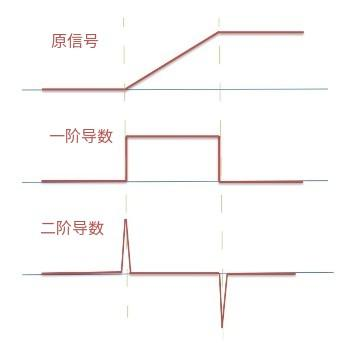1. 一阶导数在图像中产生较粗的边缘
2. 二阶导数对细节更敏感，如细线、噪声等，它提取出来的边缘更细更强(sharp)
3. 二阶导数在灰度斜坡和灰度台阶过度处会产生双边沿响应
4. 二阶导数的符号可以确定边缘的过渡是从亮到暗还是从暗到亮
5. 根据导数提取边缘之前最好对图像做平滑处理，因为导数对噪声比较敏感，尤其是二阶导数
6. 二阶导数会强化边缘和其他区域的对比度，但是也会将灰度平滑区域的噪声进行放大，使其更明显

## 三、边缘提取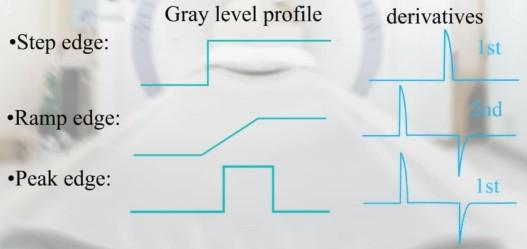### 3.1、Prewitt算子

$\begin{bmatrix} -1 & 0 & 1 \ -1 & 0 & 1 \ -1 & 0 & 1 \end{bmatrix}$ ：计算水平梯度，检测垂直边缘

$\begin{bmatrix} -1 & -1 & -1 \ 0 & 0 & 0 \ 1 & 1 & 1 \end{bmatrix}$ ：计算垂直梯度，检测水平边缘

（梯度方向跟边缘方向垂直）

### 3.2、Sobel算子

Sobel算子是在Prewitt算子的基础上进行改进，增强了中间这个位置的权重：

$\begin{bmatrix} -1 & 0 & 1 \ -2 & 0 & 2 \ -1 & 0 & 1 \end{bmatrix}$ ：计算水平梯度，检测垂直边缘

$\begin{bmatrix} -1 & -2 & -1 \ 0 & 0 & 0 \ 1 & 2 & 1 \end{bmatrix}$ ：计算垂直梯度，检测水平边缘

Sobel更强调了和边缘相邻的像素点对边缘的影响。相比较Prewitt算子，Sobel模板能够较好的抑制噪声（平滑）效果。

### 3.3、Laplacian算子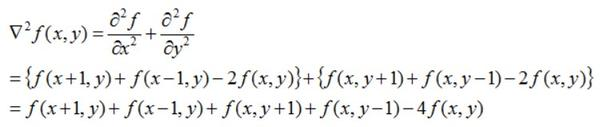$$\begin{bmatrix} 0 & 1 & 0 \ 1 & -4 & 1 \ 0 & 1 & 0 \end{bmatrix} \ \ \ \ \text or \ \ \ \ \begin{bmatrix} 0 & -1 & 0 \ -1 & 4 & -1 \ 0 & -1 & 0 \end{bmatrix}$$

$$\begin{bmatrix} 1 & 1 & 1 \ 1 & -8 & 1 \ 1 & 1 & 1 \end{bmatrix} \ \ \ \ \text or \ \ \ \ \begin{bmatrix} -1 & -1 & -1 \ -1 & 8 & -1 \ -1 & -1 & -1 \end{bmatrix}$$

## 四、平滑去噪

### 4.1、高斯滤波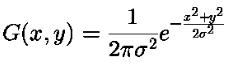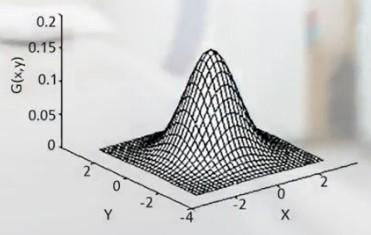## 总结

•1
•0
•274收藏

• 29

关注
• 66

获赞
• 5

精选文章

• 人脸识别方向

• 人脸识别

• 189
• 22
• 81
• 137
• 70